## Faulhaber's Formula

In a 1631 edition of Academiae Algebrae, J. Faulhaber published the general formula for the Sum of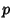th Powers of the firstPositive Integers,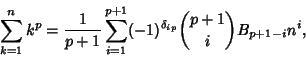(1)

where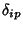is the Kronecker Delta,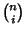is a Binomial Coefficient, and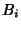is theth Bernoulli Number. Computing the sums for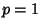, ..., 10 gives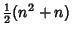(2)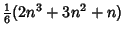(3)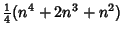(4)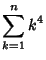(5)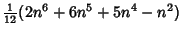(6)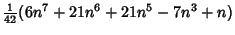(7)(8)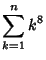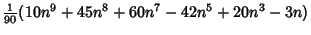(9)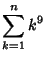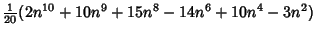(10)(11)